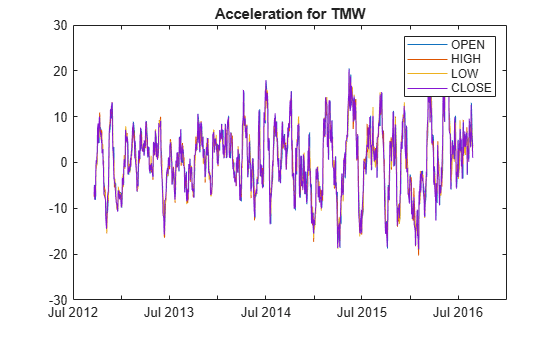Documentation

# tsmom

Momentum between times

`tsmom` has been partially removed and will no longer accept a `fints` object (`tsobj`) argument. Use a vector, matrix, `timetable`, or `table` instead for financial time series.

Use `fts2timetable` to convert a `fints` object to a `timetable` object.

## Syntax

``momentum = tsmom(Data)``
``momentum = tsmom(___,Name,Value)``

## Description

example

````momentum = tsmom(Data)` calculates the momentum of a data series with time distance of n periods.```

example

````momentum = tsmom(___,Name,Value)` adds optional name-value pair arguments. ```

## Examples

collapse all

Load the file `SimulatedStock.mat`, which provides a timetable (`TMW`) for financial data for TMW stock.

```load SimulatedStock.mat TMW.Volume = []; % remove VOLUME field momentum = tsmom(TMW); plot(momentum.Time,momentum.Variables) legend('OPEN','HIGH','LOW','CLOSE') title('Acceleration for TMW')```## Input Arguments

collapse all

Data with high, low, open, close information, specified as a vector, matrix, table, or timetable. For vector input, `Data` is a column vector. For matrix input, `Data` is an `M`-by-`N` column oriented matrix. Timetables and tables with `M` rows can contain variables named `'High'`, `'Low'`, `'Open'`, and `'Close'` (case insensitive).

Data Types: `double` | `table` | `timetable`

### Name-Value Pair Arguments

Specify optional comma-separated pairs of `Name,Value` arguments. `Name` is the argument name and `Value` is the corresponding value. `Name` must appear inside quotes. You can specify several name and value pair arguments in any order as `Name1,Value1,...,NameN,ValueN`.

Example: `momentum = tsmom(TMW,'NumPeriods',15)`

Period difference for momentum, specified as the comma-separated pair consisting of `'NumPeriods'` and a scalar positive integer.

Data Types: `double`

## Output Arguments

collapse all

Momentum series, returned with the same number of rows (`M`) and the same type (matrix, table, or timetable) as the input `Data`.

collapse all

### Momentum Series

Momentum series is the difference of the current data with the data n periods ago. By default, momentum is based on 12-period difference.

 Kaufman, P. J. The New Commodity Trading Systems and Methods. John Wiley and Sons, New York, 1987.